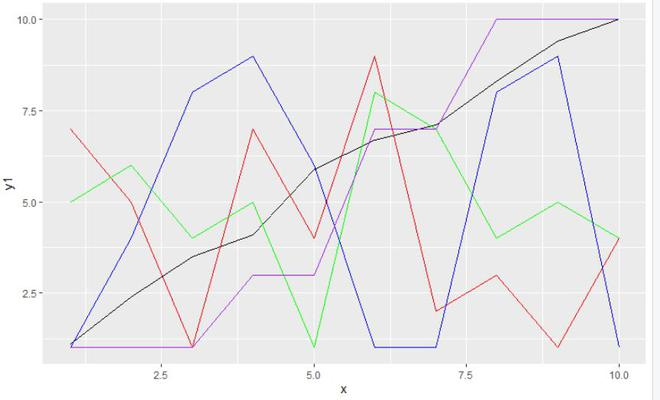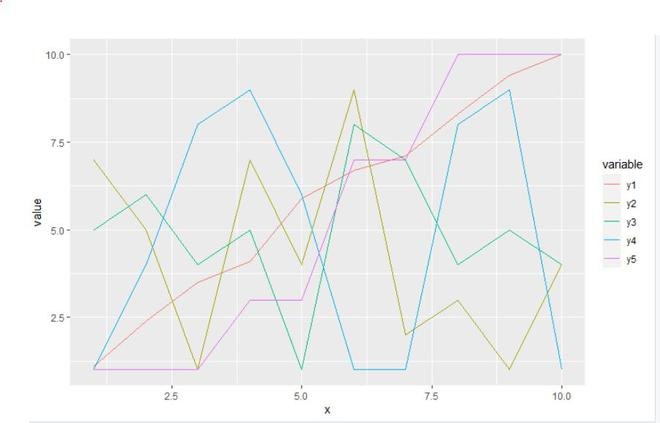# How to create a plot using ggplot2 with Multiple Lines in R ?

• Last Updated : 17 Jun, 2021

In this article, we will discuss how to create a plot using ggplot2 with multiple lines in the R programming language.

### Method 1: Using geom_line() function

In this approach to create a ggplot with multiple lines, the user need to first install and import the ggplot2 package in the R console and then call the ggplot() and the geom_line() functions in the combinations with the respected parameters as the ggplot() function will be helping to create the plot and the geom_line() function will help to create lines and when geom_line() function is called multiple times with the multiple data is return the multiples lines to the ggplot.

geom_line() function: This function is used to connect observations, ordered by x value.

Syntax: geom_line(mapping = NULL, data = NULL, stat = “identity”, position = “identity”, …)

Example:

In this example, we will be drawing five multiple lines with the different data and different colors of the line on a simple ggplot using the geom_line function from the ggplot2 package in the R programming language.

## R

 `library``(``"ggplot2"``) `` ` ` ` `gfg_data <- ``data.frame``(x = ``c``(1, 2, 3, 4, 5, 6, 7, 8, 9, 10),``                   ``y1 = ``c``(1.1, 2.4, 3.5, 4.1, 5.9, 6.7, ``                          ``7.1, 8.3, 9.4, 10.0),``                   ``y2 = ``c``(7, 5, 1, 7, 4, 9, 2, 3, 1, 4),``                   ``y3 = ``c``(5, 6, 4, 5, 1, 8, 7, 4, 5, 4),``                   ``y4 = ``c``(1, 4, 8, 9, 6, 1, 1, 8, 9, 1),``                   ``y5 = ``c``(1, 1, 1, 3, 3, 7, 7, 10, 10, 10))`` ` `gfg_plot <- ``ggplot``(gfg_data, ``aes``(x)) +  ``    ``geom_line``(``aes``(y = y1), color = ``"black"``) +``     ``geom_line``(``aes``(y = y2), color = ``"red"``) +``    ``geom_line``(``aes``(y = y3), color = ``"green"``) +``    ``geom_line``(``aes``(y = y4), color = ``"blue"``) +``    ``geom_line``(``aes``(y = y5), color = ``"purple"``)``gfg_plot`

Output:### Method 2: Using reshape2 package

In this method to create a ggplot with multiple lines, the user needs to first install and import the reshape2 package in the R console and call the melt() function with the required parameters to format the given data to long data form and then use the ggplot() function to plot the ggplot of the formatted data.

To install and import the reshape2 package in the R console, the user needs to follow the below syntax:

```install.packages("reshape2 ")
library("reshape2 ")```

melt() function: This the generic melt function. See the following functions for the details about different data structures:

Syntax: melt(data, …, na.rm = FALSE, value.name = “value”)

Parameters:

• data: Data set to melt
• …: further arguments passed to or from other methods.
• na.rm: Should NA values be removed from the data set? This will convert explicit missings to implicit missings.
• value.name: name of a variable used to store values

Example: In this example, we will be drawing five multiple lines with the different data and different colors of the line on a simple ggplot using ggplot() function and modifying the data to long data format from reshape package in the R programming language.

## R

 `library``(``"reshape2"``)  `` ` ` ` `gfg_data <- ``data.frame``(x = ``c``(1,2,3,4,5,6,7,8,9,10),``                   ``y1 = ``c``(1.1,2.4,3.5,4.1,5.9,6.7,``                          ``7.1,8.3,9.4,10.0),``                   ``y2 = ``c``(7,5,1,7,4,9,2,3,1,4),``                   ``y3 = ``c``(5,6,4,5,1,8,7,4,5,4),``                   ``y4 = ``c``(1,4,8,9,6,1,1,8,9,1),``                   ``y5 = ``c``(1,1,1,3,3,7,7,10,10,10))`` ` `data_long <- ``melt``(gfg_data, id = ``"x"``)``gfg_plot <- ``ggplot``(data_long,            ``               ``aes``(x = x,``                   ``y = value,``                   ``color = variable)) +  ``geom_line``()``gfg_plot`

Output:My Personal Notes arrow_drop_up